##### Math For Real Life For DummiesWhen using math root rules, first note that you can’t have a negative number under a square root or any other even number root — at least, not in basic calculus. Here are a couple of easy rules to begin with: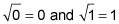But you knew that, right?

To multiply roots: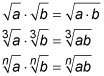To divide roots: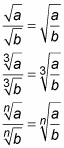To find the root of a root, you multiply the root indexes: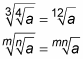If you have an even number root, you need the absolute value bars on the answer because, whether a is positive or negative, the answer is positive.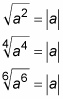If it’s an odd number root, you don’t need the absolute value bars. Thus,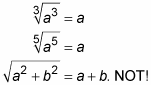Make this mistake and go directly to jail. Try solving it with numbers: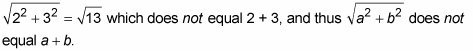Here’s a tip: any root can be converted into a power, right?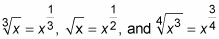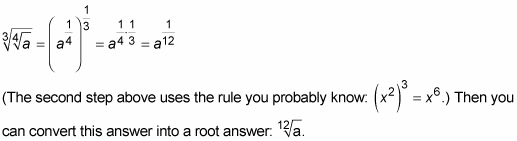So, you can often use powers to solve a problem instead of roots if you happen to forget the root rules or if you prefer working with powers to roots. For example, if you forget the above rule about multiplying the root indexes, no worries: just convert the problem into a power problem, solve the problem with the power rules (which you probably are more familiar with than the root rules), and finally, convert the power answer back into a root answer. To wit . . .